# D'Alembert principle

(diff) ← Older revision | Latest revision (diff) | Newer revision → (diff)

One of the fundamental principles of mechanical systems with constraints; it involves a general method with the aid of which the equations of motion of any mechanical system may be derived in the form of equilibrium equations of forces (in this sense d'Alembert's principle "reduces" dynamics to statics) and also determines reactions of the constraints. Formulated by J. d'Alembert  as a rule for the determination of motions (i.e. velocity vectors) of several bodies which interact by means of connecting threads or rigid rods: Each movement transmitted by the bodies is resolved into two motions — the motion which is in fact received by the bodies and some other motion, where the two motions must be such that if only the first motion is executed by the bodies, they execute it without interfering with each other, while if they execute the second motion, they will remain at rest.

The principle was successfully employed by d'Alembert and other scientists to solve a number of problems. However, the resolution of motions in accordance with the principle and the determination of the forces which must cancel each other is a difficult task. This stimulated J. Lagrange  to present a different formulation of d'Alembert's principle, based on the establishment of equilibrium between the forces and the motions produced by them but oppositely directed; this approach is close to the modern ideas on the subject. Let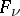andbe, respectively, the given active force and the reactions of the constraints acting on a material point with mass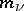moving with an acceleration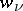; then, according to d'Alembert's principle,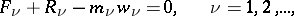(*)

i.e. at any moment of time an active force and a reaction of the constraints, applied to the moving material point, are balanced by the force of inertia —— of the point. Alternatively, if the forceis resolved into two component forces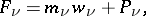the equations (*) will assume the form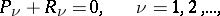i.e. at any moment of time, the "lost force"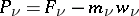is equilibrated by the force of reaction of the constraints .

How to Cite This Entry:
D'Alembert principle. Encyclopedia of Mathematics. URL: http://encyclopediaofmath.org/index.php?title=D%27Alembert_principle&oldid=46577
This article was adapted from an original article by V.V. Rumyantsev (originator), which appeared in Encyclopedia of Mathematics - ISBN 1402006098. See original article